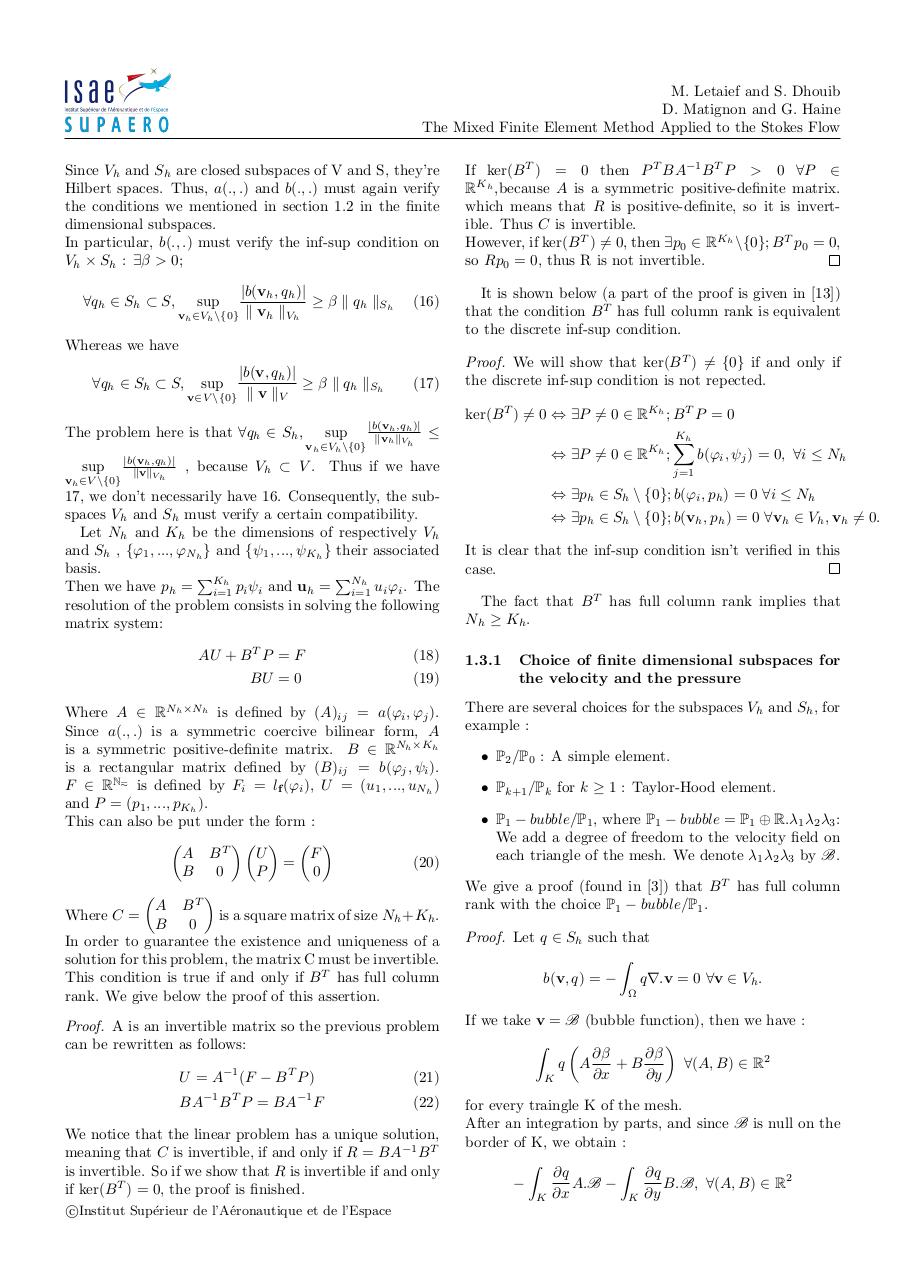# rapport (1).pdfPage 1 2 3 4 5 6 7 8

#### Text preview

M. Letaief and S. Dhouib
D. Matignon and G. Haine
The Mixed Finite Element Method Applied to the Stokes Flow
Since Vh and Sh are closed subspaces of V and S, they’re
Hilbert spaces. Thus, a(., .) and b(., .) must again verify
the conditions we mentioned in section 1.2 in the finite
dimensional subspaces.
In particular, b(., .) must verify the inf-sup condition on
Vh × Sh : ∃β &gt; 0;
∀qh ∈ Sh ⊂ S,

|b(vh , qh )|
≥ β k qh kSh
vh ∈Vh \{0} k vh kVh
sup

(16)

If ker(B T ) = 0 then P T BA−1 B T P &gt; 0 ∀P ∈
RKh ,because A is a symmetric positive-definite matrix.
which means that R is positive-definite, so it is invertible. Thus C is invertible.
However, if ker(B T ) 6= 0, then ∃p0 ∈ RKh \{0}; B T p0 = 0,
so Rp0 = 0, thus R is not invertible.
It is shown below (a part of the proof is given in )
that the condition B T has full column rank is equivalent
to the discrete inf-sup condition.

Whereas we have
|b(v, qh )|
∀qh ∈ Sh ⊂ S, sup
≥ β k qh kSh
v∈V \{0} k v kV
The problem here is that ∀qh ∈ Sh ,

sup
vh ∈Vh \{0}

sup
vh ∈V \{0}

|b(vh ,qh )|
kvkVh

(17)

|b(vh ,qh )|
kvh kVh

Proof. We will show that ker(B T ) 6= {0} if and only if
the discrete inf-sup condition is not repected.
ker(B T ) 6= 0 ⇔ ∃P 6= 0 ∈ RKh ; B T P = 0

⇔ ∃P 6= 0 ∈ RKh ;

, because Vh ⊂ V . Thus if we have

17, we don’t necessarily have 16. Consequently, the subspaces Vh and Sh must verify a certain compatibility.
Let Nh and Kh be the dimensions of respectively Vh
and Sh , {ϕ1 , ..., ϕNh } and {ψ1 , ..., ψKh } their associated
basis.
PKh
PNh
Then we have ph = i=1
pi ψi and uh = i=1
ui ϕi . The
resolution of the problem consists in solving the following
matrix system:
AU + B T P = F

(18)

BU = 0

(19)

Where A ∈ RNh ×Nh is defined by (A)ij = a(ϕi , ϕj ).
Since a(., .) is a symmetric coercive bilinear form, A
is a symmetric positive-definite matrix. B ∈ RNh ×Kh
is a rectangular matrix defined by (B)ij = b(ϕj , ψi ).
F ∈ RNh is defined by Fi = lf (ϕi ), U = (u1 , ..., uNh )
and P = (p1 , ..., pKh ).
This can also be put under the form :

   
U
F
A BT
=
(20)
P
0
B
0


A BT
is a square matrix of size Nh +Kh .
Where C =
B
0
In order to guarantee the existence and uniqueness of a
solution for this problem, the matrix C must be invertible.
This condition is true if and only if B T has full column
rank. We give below the proof of this assertion.
Proof. A is an invertible matrix so the previous problem
can be rewritten as follows:
U = A−1 (F − B T P )
BA

−1

T

B P = BA

−1

F

(21)
(22)

We notice that the linear problem has a unique solution,
meaning that C is invertible, if and only if R = BA−1 B T
is invertible. So if we show that R is invertible if and only
if ker(B T ) = 0, the proof is finished.
c
Institut
Sup´erieur de l’A´eronautique et de l’Espace

Kh
X

b(ϕi , ψj ) = 0, ∀i ≤ Nh

j=1

⇔ ∃ph ∈ Sh \ {0}; b(ϕi , ph ) = 0 ∀i ≤ Nh
⇔ ∃ph ∈ Sh \ {0}; b(vh , ph ) = 0 ∀vh ∈ Vh , vh 6= 0.
It is clear that the inf-sup condition isn’t verified in this
case.
The fact that B T has full column rank implies that
Nh ≥ K h .
1.3.1

Choice of finite dimensional subspaces for
the velocity and the pressure

There are several choices for the subspaces Vh and Sh , for
example :
• P2 /P0 : A simple element.
• Pk+1 /Pk for k ≥ 1 : Taylor-Hood element.
• P1 − bubble/P1 , where P1 − bubble = P1 ⊕ R.λ1 λ2 λ3 :
We add a degree of freedom to the velocity field on
each triangle of the mesh. We denote λ1 λ2 λ3 by B.
We give a proof (found in ) that B T has full column
rank with the choice P1 − bubble/P1 .
Proof. Let q ∈ Sh such that
Z
b(v, q) = −
q∇.v = 0 ∀v ∈ Vh .

If we take v = B (bubble function), then we have :


Z
∂β
∂β
q A
+B
∀(A, B) ∈ R2
∂x
∂y
K
for every traingle K of the mesh.
After an integration by parts, and since B is null on the
border of K, we obtain :
Z
Z
∂q
∂q

A.B −
B.B, ∀(A, B) ∈ R2
∂x
∂y
K
K